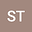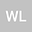loading page

Lower and upper bounds of Dirichlet eigenvalues for Grushin type degenerate elliptic operators in weighted divergence form with a potential
••• Shenyang Tan,
• Wenjun Liu
Shenyang Tan
Nanjing University of Information Science and Technology
Author ProfileWenjun Liu
Nanjing University of Information Science and Technology
Author Profile## Abstract

In this paper, we consider the estimates of Dirichlet eigenvalues for Grushin type degenerate elliptic operator in weighted divergence form with a potential $-{\rm div}_{G}(A\nabla_{G})+\langle A\nabla_{G}\phi,\nabla_{G}\rangle-V$. Using the method of Fourier transformation, we get precise lower bound estimates for the eigenvalues. Then, through the way of trail function, we obtain Yang-type inequalities which give upper bounds of eigenvalues.